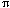Name: mara frost Who is asking: Student Level: Middle Question: what is the formula to find the circumference of a circle, or if there is no formula, how do you find the circumference of a circle? Thank you Hi Mara, An amazing fact about a circle is that if you measure its circumference and divide by its diameter you always get the same answer. It makes no difference how large the circle is the answer is always 3.14159... This number is so important it gets its own symbol,. If you call the circumference c and the diameter d then this is your formula, c/d =, or rewriting it c =d.    Often you measure the radius, r, of a circle rather than its diameter and since d = 2 r the expression for the circumference is most often seen as c = 2r. Cheers, Penny Go to Math Central